Class 10 RD Sharma Solutions – Chapter 10 Circles – Exercise 10.2 | Set 1

• Last Updated : 05 May, 2021

Question 1. If PT is a tangent at T to a circle whose centre is O and OP = 17 cm, OT = 8 cm. Find the length of the tangent segment PT.

Solution: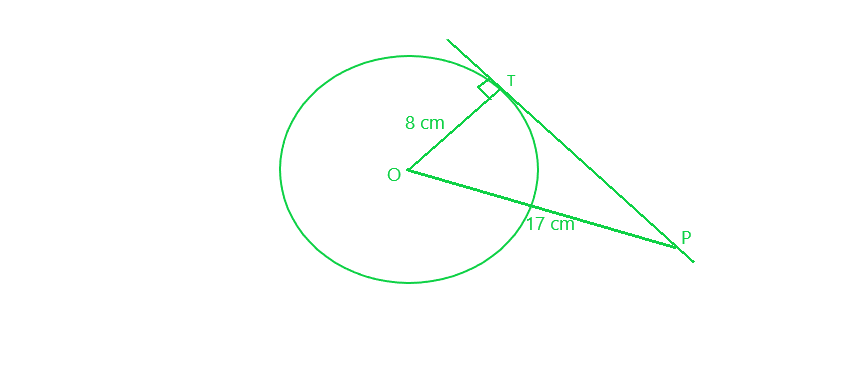PT is the tangent to the circle with centre O, at T

=> PT⊥ OT

OP = 17 cm

Therefore, In right ∆OPT, by applying Pythagoras Theorem:

OP² = OT² + PT²

=> (17)² = (8)² + PT²

=> 289 = 64 + PT²

=> PT² = 289 – 64 = 225 = (15)²

PT = 15 cm

Question 2. Find the length of a tangent drawn to a circle with radius 5 cm, from a point 13 cm from the centre of the circle.

Solution: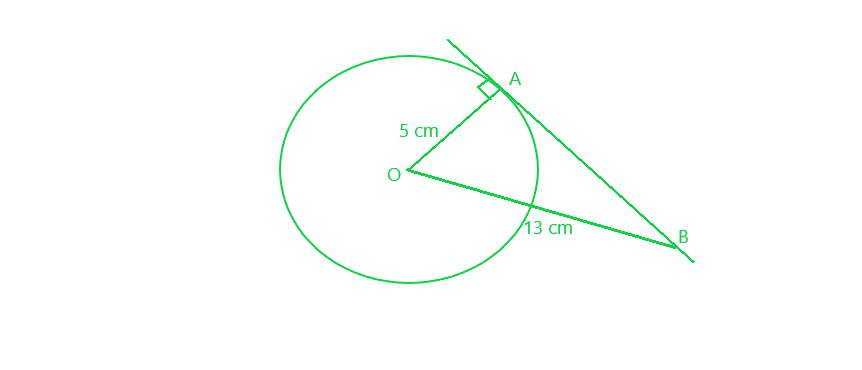From a point P outside the circle with centre O, let us draw a tangent PT to the circle

OB = 13 cm

OA ⊥ BA

Therefore, In right ∆OPT, by applying Pythagoras Theorem

OB² = OA² + BA²

(13)² = (5)² + BA²

=> 169 = 25 + BA²

=> BA² = 169 – 25 = 144 = (12)²

BA = 12 cm

Question 3. A point P is 26 cm away from the centre O of a circle and the length PT of the tangent drawn from P to the circle is 10 cm. Find the radius of the circle.

Solution: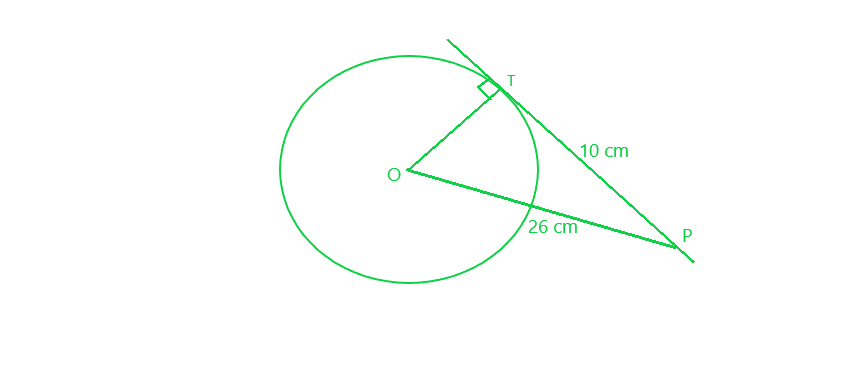From a point P outside the circle with centre O, let us draw a tangent PT to the circle of radius OT

OP = 26 cm

PT = 10 cm

Therefore, In right ∆OPT, by applying Pythagoras Theorem:

OP² = OT² + PT²

=> (26)² = OT² + (10)²

=> 676 = OT² + 100

=> 676 – 100 = OT²

=> OT² = 576 = (24)²

Hence, radius of the circle = 24 cm

Question 4. If from any point on the common chord of two intersecting circles, tangents be drawn to the circles, prove that they are equal.

Solution: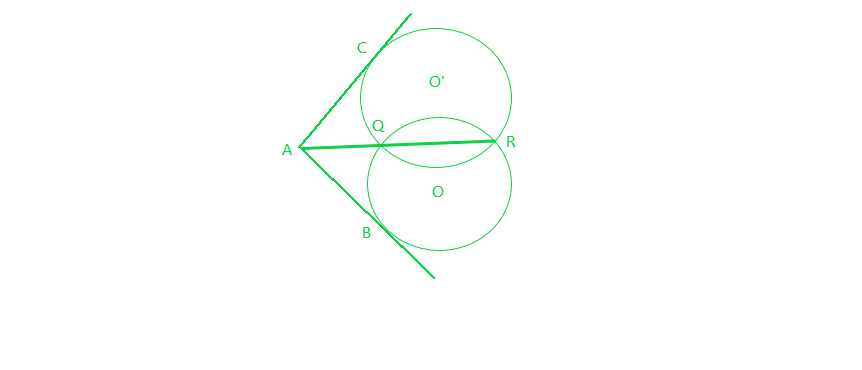Given:

QR is the common chord of two circles intersecting each other at Q and R

A is a point on RQ when produced.

From A, AB and AC two tangents are drawn to the circles with centres O and O’ respectively.

To prove : AB = AC

Proof: AB is the tangent and AQR is the secant to the circle with centre O

=> AB² = AQ x AR     (1)

Similarly, AC is the tangent and AQR is the secant to the circle with centre O’

=> AC² = AQ x AR     (2)

From (1) and (2)

AB² = AC²

AB = AC

Hence, proved.

Question 5. If the sides of a quadrilateral touch a circle, prove that the sum of a pair of opposite sides is equal to the sum of the other pair.

Solution: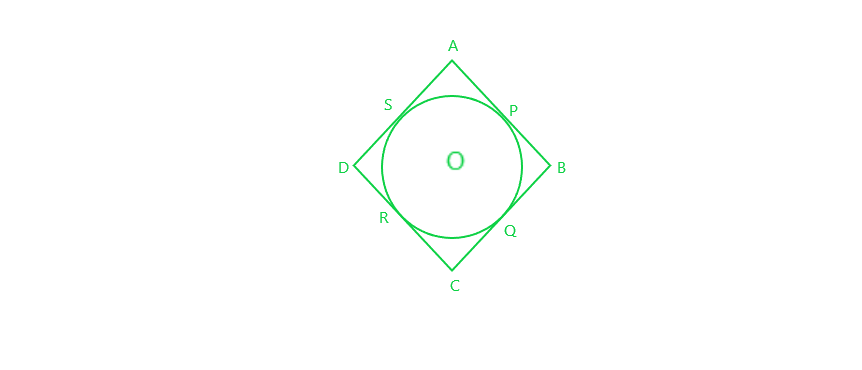Given: The sides of a quadrilateral ABCD touch the circle at P, Q, R and S respectively

To prove : AB + CD = AP + BC

Proof : AP and AS are the tangents to the circle from A

=> AP = AS     (1)

Similarly, BP = BQ     (2)

and CR = CQ     (3)

and DR = DS     (4)

Adding, all the four equation we get:

AP + BP + CR + DR = AS + BQ + CQ + DS

=> (AP + BP) + (CR + DR) = (AS + DS) + (BQ + CQ)

=> AB + CD = AD + BC

Hence, proved.

Question 6. Out of the two concentric circles, the radius of the outer circle is 5 cm and the chord AC of length 8 cm is a tangent to the inner circle. Find the radius of the inner circle.

Solution: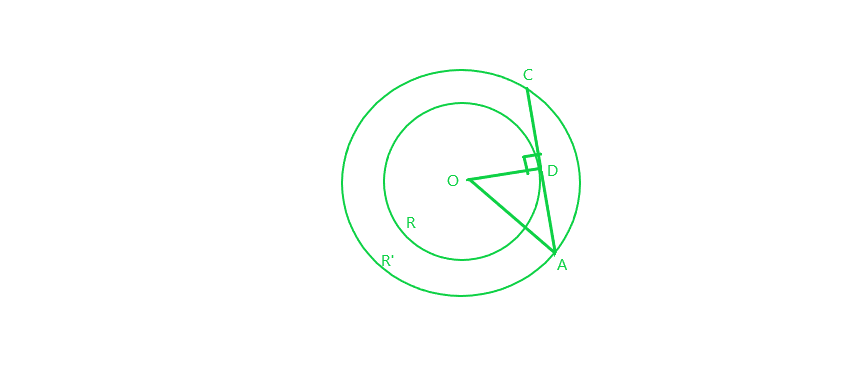Let R and R’ be the two circles having same centre O. AC is a chord which touches the R at point D

Now, OD ⊥ AC

AD = DC = 4 cm     [perpendicular line OD bisects the chord at D]

OA = 5 cm     [given]

Therefore, In right-angled ∆AOD, by applying Pythagoras theorem:

=> OD² = 5² – 4² = 25 – 16 = 9

=> OD = 3 cm

Thus, Radius of the inner circle OD = 3 cm

Question 7. A chord PQ of a circle is parallel to the tangent drawn at a point R of the circle. Prove that R bisects the arc PRQ.

Solution:Given: Chord PQ is parallel to the tangent at R.

To prove : R bisects the arc PRQ (i.e., PR=QR)

Proof:

∠1 = ∠2       [alternate interior angles]   (1)

∠1 = ∠3      [angle between tangent and chord is equal to angle made by chord in alternate segment]   (2)

=> ∠2 = ∠3     [from (1) and (2)]

=> PR = QR      [sides opposite to equal angles are equal]

So, R bisects PQ

Question 8. Prove that a diameter AB of a circle bisects all those chords which are parallel to the tangent at the point A.

Solution: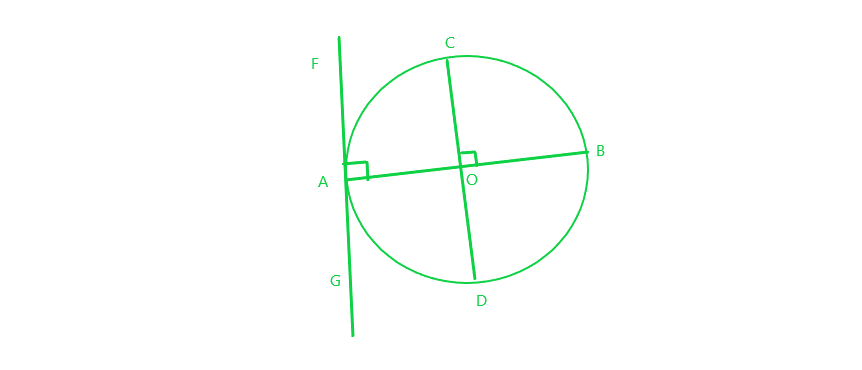Given:

AB is a diameter of the circle.

A tangent is drawn from point A.

A chord CD parallel to the tangent FAG.

To prove: AB bisect CD and hence all the chords parallel to the tangent at the point A.

Proof: CD is a chord of the circle and OA is a radius of the circle.

∠FAO = 90°     [Tangent at any point of a circle is perpendicular to the radius through the point of contact]

∠COB = ∠FAO      [corresponding angles]

=> ∠COB = 90°

Thus, AB bisects CD     [perpendicular from centre of circle to chord bisects the chord]

Similarly, the diameter AB bisects all the chords which are parallel to the tangent at the point A.

Question 9. If AB, AC, PQ are the tangents in the figure, and AB = 5 cm, find the perimeter of ∆APQ.

Solution:AB, AC and PQ are the tangents to the circle as shown in the figure above and AB = 5 cm

PB and PR are the tangents to the circle from the same point P

=> PB = PR

Similarly, QC and QR are the tangents from the same point Q

=> QC = QR

Also, AB and AC are the tangents from A

=> AB = AC = 5 cm

Now perimeter of ∆APQ

= AP + PQ + AQ

= AP + PR + RQ + AQ

= AP + PB + QC + AQ      {because PB = PR and QC = QR}

= AB + AC

= 5 cm + 5 cm

=10 cm

So, Perimeter of the triangle APQ is 10 cm

Question 10. Prove that the intercept of a tangent between two parallel tangents to a circle subtends a right angle at the centre.

Solution: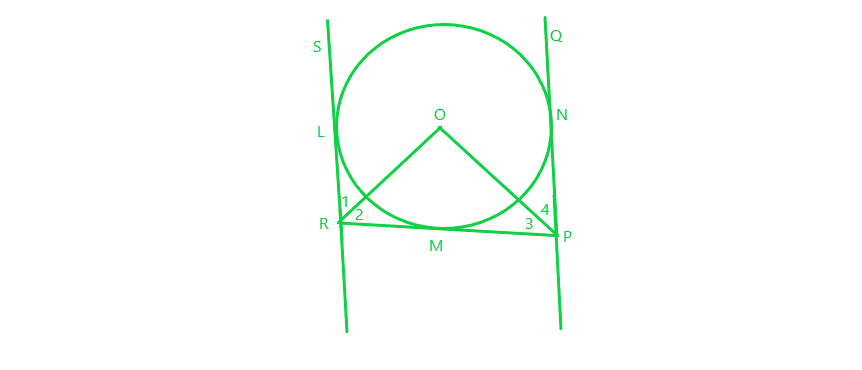Given:

PQ and RS are parallel tangents of a circle

RMP is the intercept of the tangent between PQ and RS

RS and PQ are joined at the centre of the circle O

To prove: ∠ROP = 90°

Proof:

Since RL and RM are tangents from R and RO is joined at the centre of the circle, it will bisect the angle

=> ∠1 = ∠2     (1)

Similarly, PM and PN are tangents from P and PO is joined at the centre of the circle, it will bisect the angle

=> ∠4 = ∠3     (2)

Adding (1) and (2), we get:

∠1+∠4 = ∠2+∠3     (3)

Also, ∠LRP + ∠RPQ = 180°     [co-interior angles]

=> ∠1+∠2+∠3+∠4 = 180°

=> ∠2+∠2+∠3+∠3 = 180°    [using (3)]

=> ∠2+∠3 = 90°     (4)

Also, in ∆POR:

∠2+∠3+∠O = 180°     [sum of all the angles of triangle is 180°]

=> 90° + ∠O = 180°

=> ∠O = 90°

So, ∠ROP = 90°

Hence Proved

My Personal Notes arrow_drop_up